# Mathamania discussion

32 views
geometry

message 1: by (new)

message 4: by (last edited Jan 28, 2009 12:54PM) (new)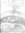Okay, I'm going to give it a try. Not promising it will make sense... =D

Converse of the Triangle Proportionality Theorem:
If a line divides two sides of a triangle
proportionally, then it is parallel to the third side.

Proof:

In order for a line to divide two sides a triangle proportionally, it must create two overlapped similar triangles. For the triangles to be similar (proportional) they're angles must be equal. If all the angles are equal then the line must be parallel to the third side of the triangle.

For example: In order to divide a right triangle proportionally, the line must create another 90 degree angle (along with the other angles). The only way to get a 90 degree angle is to have a straight line intersect a straight line. The intersecting straight line then, must be parallel to one or the other straight lines of the right triangle.

message 5: by Joon, Math genius (new)

This is going to be fun.

message 6: by (new)

message 7: by (new)Oh, and did I do any good explaining it? It's really hard without a diagram.

message 8: by Joon, Math genius (last edited Jan 28, 2009 06:40PM) (new)

Your explanation was perfect. Oh, and to answer your question, we are going to have fun doing super hard problems.

message 9: by (new)Yay! Thanks Joon! =D

Yes, we are going to have so much fun doing really hard problems! I thrive on puzzles! The only bad part is that, when faced with a puzzle/problem, it sort of takes over my brain (or is, at least, annoyingly naggy) until I know the answer. :)

message 10: by (last edited Jan 29, 2009 10:01AM) (new)Alright, here's another one:

The radius of the circle is 12cm. There is an angle like this < (angle ABC) in the circle, but it does NOT pass through the center of the circle and who's inner angle is 40 degrees. Find the length of arc ABC. Round the answer to the nearest hundredth. (Again, this would be much easier if I was able to post a diagram.)

message 12: by (new)

message 13: by (new)

message 14: by (new)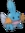Elaine, what grade are you in? and what mathe course do you take in school?
yejin--how was spicewood?

message 15: by (new)

message 16: by (new)Why? Sorry, but I don't feel comfortable sharing that information.

message 17: by Joon, Math genius (new)

Not all people like to tell information. Some people like to stay reserved. Oh, and I thought that the Spicewood thing was okay. I had to play Pink Panther about 25 times.

message 18: by (new)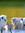me, too. The songs and i memorized every single word we said even other people's words in woodwind quintet.

message 21: by (new)Yep.

Definition for Geometry:

the branch of mathematics dealing with the properties, measurements, and relationships of points, lines, planes, and solids.

Sorry, I was curious. =)

message 22: by Joon, Math genius (new)

Yeah, most of it deals with shapes, lines, degress, etc. It is not easy if you are not a good visualizer. (Like me.)

message 23: by (new)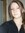Oooo in england we don't really categorize them into geometry, algebra ect x

message 24: by Joon, Math genius (new)

Then how does your math system go?

message 25: by (new)We have different sections. This section i'm doing now has algebra, quadratic graphs, Surds, and some more stuff. Do you do GCSEs?

message 26: by (new)

message 27: by (new)surds are like

√20 is a surd because the number is not whole.

but say √8 simplified is √4 x √2 which is written like this: 2√2

It's hard to explain on a computer.

message 28: by (new)Nope, I get it. That's interesting that it's its own section.

message 29: by Joon, Math genius (new)

That is cool. That makes perfect sense.

message 30: by (new)Yeah! And you like add, divide, subtract-ect them.

But for my non-calculator exam I had to find √20
I was like huh??

message 31: by (new)Elaine wrote: "Alright, here's another one:

The radius of the circle is 12cm. There is an angle like this < (angle ABC) in the circle, but it does NOT pass through the center of the circle and who's inner angle is 40 degrees. Find the length of arc ABC. Round the answer to the nearest hundredth. (Again, this would be much easier if I was able to post a diagram.)

No one has answered my question yet!!!

message 32: by (new)Elaine wrote: "Elaine wrote: "Alright, here's another one:

The radius of the circle is 12cm. There is an angle like this

No one has answered my question yet!!!

"

i agree with you...i don't rly get the question b/c idk how the diagram looks like.....i wish we could make a diagrams on computers

message 33: by (last edited May 17, 2010 08:46PM) (new)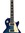First should know that if
I used 80 because that is the degree measure of arc ABC, and if the radius is 12cm, then the circumference is (diameter times pi) 75.36.

Then you can cross multiply to get 80(75.36)=360x. 6028.8=360x.

x (or the length of the arc) = 16.75 cm. Am I correct?

message 34: by (new)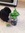OK time for aa coordinate geometry question....

Easy one to start:

Find the midpoint of the line AB when A(3,8) and B (9,-6).